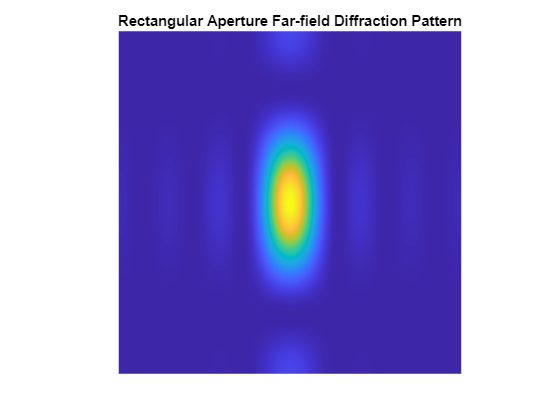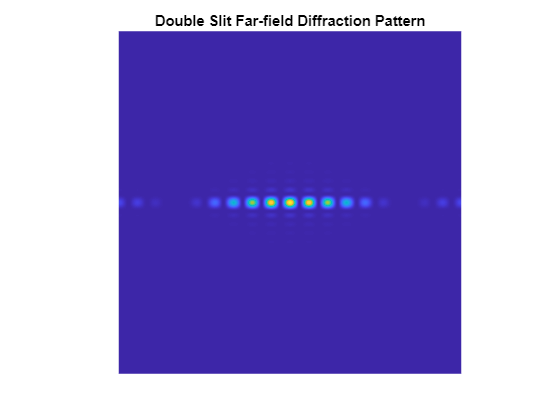# Use FFT2 on the GPU to Simulate Diffraction Patterns

This example uses Parallel Computing Toolbox™ to perform a two-dimensional Fast Fourier Transform (FFT) on a GPU. The two-dimensional Fourier transform is used in optics to calculate far-field diffraction patterns. These diffraction patterns are observed when a monochromatic light source passes through a small aperture, such as in Young's double-slit experiment.

### Define the Coordinate System

Before you simulate the light that has passed through an aperture, define a coordinate system. To get the correct numerical behavior for calling `fft2`, arrange `x` and `y` so that the zero value is in the correct place.

`N2` is half the size in each dimension.

```N2 = gpuArray(1024); [gx,gy] = meshgrid(-1:1/N2:(N2-1)/N2);```

### Simulate the Diffraction Pattern for a Rectangular Aperture

Simulate the effect of passing a parallel beam of monochromatic light through a small rectangular aperture. The two-dimensional Fourier transform describes the light field at a large distance from the aperture. Start by forming `aperture` as a logical mask based on the coordinate system. The light source is a double-precision version of the aperture. The far-field light signal is found using `fft2`.

```aperture = (abs(gx)<4/N2).*(abs(gy)<2/N2); lightsource = double(aperture); farfieldsignal = fft2(lightsource);```

### Display the Light Intensity for a Rectangular Aperture

Calculate the far-field light intensity from the magnitude squared of the light field. Use `fftshift` to aid visualization.

```farfieldintensity = real(farfieldsignal.*conj(farfieldsignal)); imagesc(fftshift(farfieldintensity)); axis("equal"); axis("off"); title("Rectangular Aperture Far-field Diffraction Pattern");```### Simulate Young's Double-Slit Experiment

One of the most famous experiments in optics is Young's double-slit experiment which shows light interference when an aperture comprises two parallel slits. A series of bright points is visible where constructive interference takes place. Form the aperture representing two slits. Restrict the aperture in the `y` direction to ensure that the resulting pattern is not entirely concentrated along the horizontal axis.

```slits = (abs(gx)<=10/N2).*(abs(gx)>=8/N2); aperture = slits.*(abs(gy)<20/N2); lightsource = double(aperture); farfieldsignal = fft2(lightsource);```

### Display the Light Intensity for Young's Double-Slit

Calculate and display the intensity as before.

```farfieldintensity = real(farfieldsignal.*conj(farfieldsignal)); imagesc(fftshift(farfieldintensity)); axis("equal"); axis("off"); title("Double Slit Far-field Diffraction Pattern");```